﻿ Einstein special relativity paradox wrong !

# Einstein is wrong.

## Einstein relativity is the biggest scam.

### [ Einstein relativity includes "true" paradoxes. ]

(Fig.1)  Einstein is wrong in special relativity.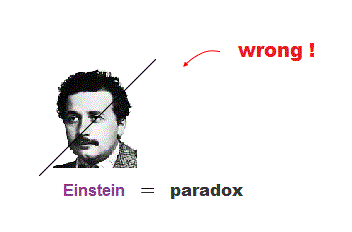Though the media and universities try to deify Einstein, his relativistic theory is filled with fatal flaws, so wrong.

In fact, almost all textbooks and the media are hiding true paradoxes of Einstein reltivity so desperately that even anti-relativists don't know them.

Ordinary people should not be misled by "dummy" relativistic paradoxes such as twin paradox, which never happens within special relativity.

Here we explain one of true paradoxes of special relativity.
If the relativity includes true paradoxes, it is destroying all strudents' careers now.

It means if students and dropouts sue unievrsities for deceiving them by wrong theory, they could surely win back their money paid as tuition.

## How was Einstein relativity born ?

### [ Special relativity was derived from constant light speed "c". ]

(Fig.2)  Light and observer K' are moving at c and v.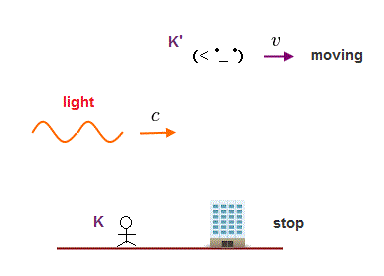Einstein special relativity is based on Lorentz transformation.
First, we explain how this special relativity was born.

Suppose the light travels at a speed of "c", and an observer K' is moving at a speed of "v" in the same direction.

All other things such as an observer K and buildings stop.

## From the viewpoint of moving observer K'

### [ Moving observer K' sees the light traveling slower at c - v. ]

(Fig.3)  K' sees other things moving in the opposite direction.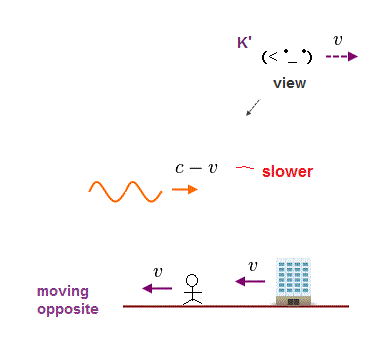How do other things look from the viewpoint of the observer K' moving at a speed of "v" ?

Of course, all other ( stationary ) things appear to be moving in the opposite direction at "v", seen from K'.

How about the light speed c ?
The light speed c naturally appears slower to c - v instead of c, seen from K'

## Light speed is always "c" for any observers ?

### [ Seen by moving observer K', the light speed remains "c" ? ]

(Fig.4)  Both moving K' and stationary K see the light traveling at "c" ?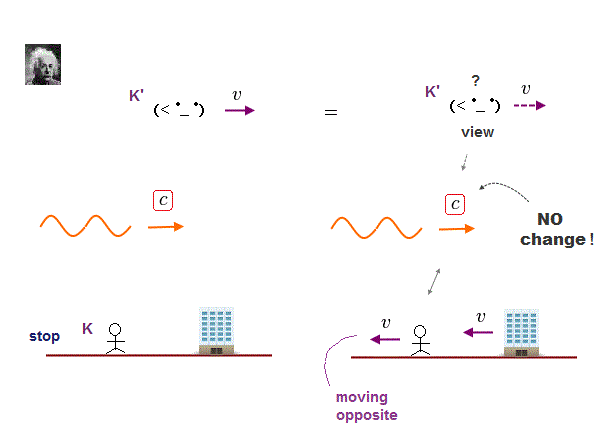Einstein special relativity is unrealistic.
It claims the light speed always becomes "c" seen from any observers.

Even when the light speed is "c" seen from the stationary K, it appears to travel at the same speed "c" seen from the moving observer K'.

This Einstein idea is ridiculous and nonsense.

Because it's natural that relative velocity seen from an observer changes depending on the observer's velocity.

## Einstein's ridiculous delusion.

### [ Even when light and an observer are moving at the same c, ]

(Fig.4')  Light speed appears to remain "c", Einstein claimed.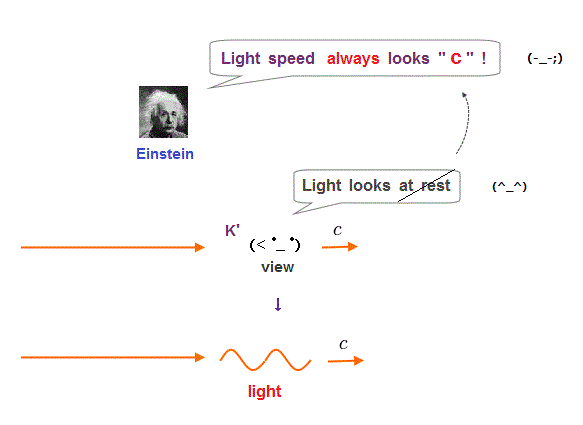In Fig.4', the light and an observer are moving at the same speed of "c" in the same direction.

From the realistic viewpoint, this light appears to stop seen from this observer.  But Einstein rejected this common sense.

He claimed even this observer sees the light moving at the same relative velocity "c", which is independent of the oberver's motion.

In fact, this Einstein's false logic was caused by misinterpreting Michelson-Morley experiment.

If we admit some "medium" moving with the earth, Einstein unrealistic relativity is unnecessary.

## From the viewpoint of stationary observer K

### [ Seen by stationary K, the light travels at a speed "c". ]

(Fig.5)  Light is emitted from the source ( x = 0 ) at time t = 0.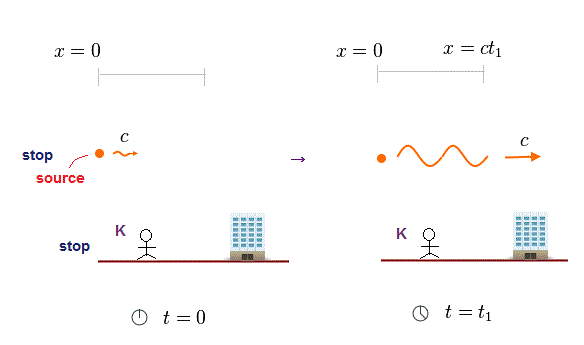Suppose the light is emitted from its source at x = 0 at time t = 0.
The light travels at a speed of "c" seen from a stationary observer K.

After the time t1 has passed, the light wavefront arrives at x = ct1.

## Seen from moving observer K'  -- Real case.

### [ Light looks slower at c - v than c. ]

(Fig.6)  Seen by K' moving at a speed of v, light looks slower.Fig.6 is a realistic case when seen from an observer K' moving at a speed "v" in the same direction.

The light head appears to travel at c - v, which is slower than "c", seen from K', because K' observer is also moving in the same direction.

The light source fixed at x = 0 is moving in the opposite direction at v, seen from K'.

## Seen by moving observer K'  -- Einstein case.

### [ Clock of K' ticks differently from stationary K ! ]

(Fig.7)  Clock time of K' lapses differently from clock K !?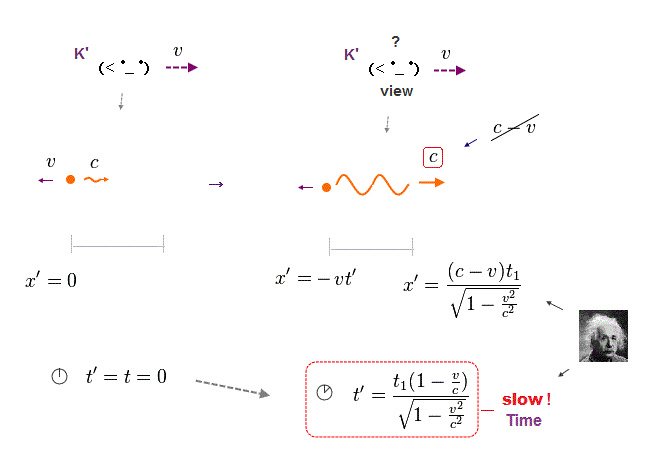As I said, Einstein relativity adopts unrealistic idea.
Relative velocity of the light seen from any observers is always "c".

To achieve this impossible logic, we have to discard our common sense.

Einstein claimed the clock time of moving K' moves on at a different pace from stationary K !

Suppose the light is emitted from its source ( fixed at x = 0 ) at time t = t' = 0.

After time t1 has passed seen from stationary K, the clock time (= t' ) of moving K' becomes different (= slower ) from t1 !

So in Einstein relativity, the time (= t' ) and space (= x' ) in different observers change in a completely different way !  This is ridiculous.

## As a result, the light speed remains "c" ?

### [ Strange changes in time and space keep the constant light speed c. ]

(Fig.8)  The light speed seen from the moving K' remains "c" ?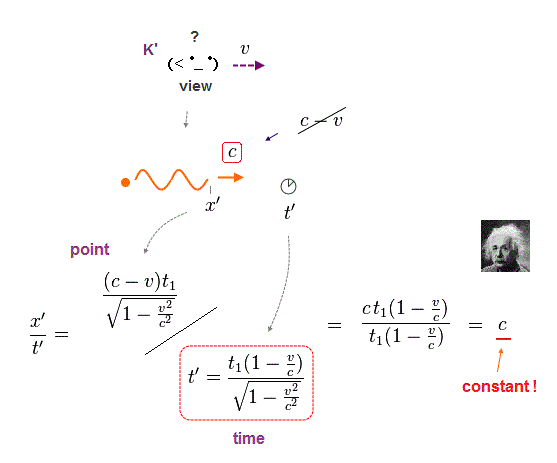According to Einstein relativity, the position (= x' ) and the clock time (= t' ) at the light wavefront become distorted, seen from the moving observer K'.

Dividing the point coordinate of the light head by the clock time, you find the relative light speed remains "c" (= x'/t' ).

This absurd logic is Einstein relativity.

## Lorentz transformation

### [ Einstein spacetime obeys Lorentz transformation. ]

(Fig.9)  Spacetimes in stationary (= t, x ) and moving (= t', x' ) observers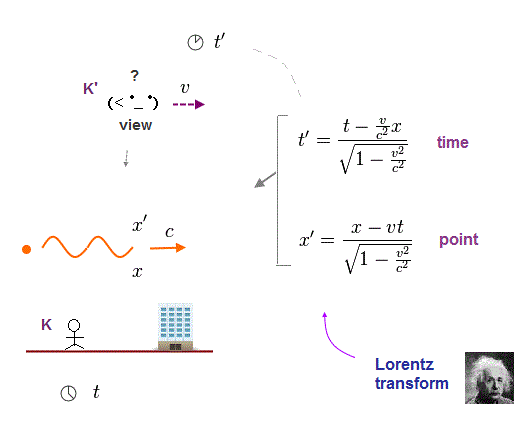This change in Einstein's spacetime depending on observer's motion obeys Lorentz transformation.

The space coordinate (= x ) and the clock time (= t ) seen from stationary K observer.

The space coordinate (= x' ) and the clock time (= t' ) seen from K' observer moving at v in x direction.

This strange world is Einstein relativity.

## Three rockets are launched at the same time.

### [ Three rockets are launced simultaneously from A, B, C sites. ]

(Fig.10)  Three rockets are rising at the same speed.Three identical rockets are launched at the same time from different A, B, C sites in Fig.10.

And these rockets are going up at the same speed.
Stationary observer K sees them.

## Seen by observer K' moving at a speed v

### [ Launching times of A, B, C rockets are different !? ]

(Fig.11)  C rocket is first launched, seen from K' !According to Einstein relativity, the launching times of A,B,C rockets look different, seen from the observer K' moving at a speed v to the right.

Surprisingly, Einstein claims the rocket C is first launched, next B, and A rocket is launched lastly !

When the stationary observer K sees these same rockets, they are launched at the same time.

So in Einstein relativity, the clock times at different positions (= A, B, C ) are moving on differently !

## Why K and K' see different launching times ?

### [ Time t' = 0 in K' are different (= t ) at different positions x in K. ]

(Fig.12)  K' observer sees different times ( t = 0, v/c2 ) of K at time t' = 0.Here we explain why this strange phenomenon happens using Lorentz transformation.

Seen from the stationary observer K, both A and B are rising at the same speed at the same altitude.

Fig.12 left shows the altitudes of two rockets at times t = 0 and t = v/c2.  Of course, these two rockets are always at the same altitude, seen from K.

But seen from observer K moving at v, the rocket B appears to be launched earlier than A.  The launching time difference is v/c2.

## At time t' = 0 seen from K'

### [ The clock times are different at different positions seen from K. ]

(Fig.13)  Different sets of ( t, x ) give t' = 0.When the clock time seen by moving observer K' is t' = 0, what is happening seen from the stationary observer K ?

The x coordinates of A and B rockets are x = 0 and x = 1, respectively.  We need to insert different t (= 0 and v/c2 ) into this to get the same t' = 0.

This means when the clock time seen from K' is t' = 0, the clock times seen from K are different in different positions x = 0, 1.

## Rigid straight rod is moving upward.

### [ Rigid straight rod is rising, keeping it horizontal. ]

(Fig.14)  The rod positions at times t = 0, 1, 2.A rigid straight rod is going upward along the rail.
The rod direction keeps horizontal.

The positions of the rod at times t = 0, 1 and 2 are shown in Fig.14.
X axis is horizontal.  Stationary observer K sees the rod.

## The rod is bent seen by moving observer !

### [ Einstein claims the rigid rod looks bent askew seen from K'. ]

(Fig.15)  When the observer moving at v to the right sees the rod, ..How does the rod look, when the observer K' moving at a speed v in the x direction sees it ?

Einstein relativity claims the horizontal rod seen from stationary K appears to be bent obliquely to upper right seen from K'.

Because as I said, different t (= 0 and v/c2 ) at different x (= 0, 1 ) give the same t' = 0.

## Straight rod is moving along a square rail.

### [ Straight rigid rod is moving along the rail keeping horizontal. ]

(Fig.16)  Seen by a stationary observer K.A straight rigid rod is moving along a square rail with rounded corners.
We move this rod, keeping its direction horizontal.

A stationary observer K sees the motion of this rod.

## The ridig rod is bent seen from K' !

### [ Seen by moving ovserver K', the rigid rod is bent. ]

(Fig.17)  Moving observer K' sees the rods at different times t.How does the rigid straight rod look, seen from an observer K' moving to the right ?

As I said in this and this, the observer K' sees a "bent" rod, though it is originally straight.

The problem is this rigid straight rod is bent very complexly when the rod is moving in two directions.

The "right" part of this rod in K' shows the "future" event in K (= t ).
The "left" part of this rod in K' shows the "past" event in K.

## Straight rod seen by moving observer K'

### [ Einstein claims the rigid straight rod is "bent" complexly ! ]

(Fig.18)  Oblieque → crooked → straight, seen from K'As I said, the right part of the rod seen from K' shows the "future" (= larger t ) event seen from K.

So when two directions ( upward → right ) are involved, the rod appears to be bent very complexly seen from the moving observer K'.

Of course, this rod is supposed to be "rigid" and very hard, so changing the shape of rigid body is impossible and nonsense.

## A block changes the rod's shape without touching it ?

### [ The moment we put a block at the corner, the rod's shape changes ? ]

(Fig.19)  Inserting a block changes the rod's future without touching ?The serious problem happens when we put a block on the corner, before the rod has Not arrived at the corner yet.

If we put a block on the way of the rod, this rod cannot turn right at the corner of the rail, which changes "future" event (= shape ) of the rod.

So the moment we put a block at the corner, the rod shape changes suddenly, though the block does NOT touch the rod at all !

This is clearly a paradox, and shows Einstein relativity is wrong.

## Change the rod's shape without touching it !?

### [ An inserted block can change the rod's shape without touching it ? ]

(Fig.20)  A block →Rod's future position change →Rod's shape changes.When we put a block at the corner, before the rod hasn't arrived there, the rod cannot turn right, so the rod's future position changes suddenly.

It means the rod's shape changes, the moment we put a block !
This phenomenon caused by Einstein relativity is ridiculous and unrealistic.

Because the inserted block can change the rigid rod's shape, without touching the rod !

This "true" paradox in Einstein relativity is caused by clock time change by Lorentz transformation.

The rod can predict its future event and bend its shape in advance, before it does NOT know the place where the rod can turn right, like a "psychic".

## A block seen from stationary K

### [ The rod cannot turn to the right at the corner. ]

(Fig.21)  When a block is put, the rod keeps moving upward.How does it look seen from stationary observer K, when a block is put at the corner ?

After a block is put, the rod cannot turn to the right at the corner.
So it keeps moving upward.

So the insertion of a block affects the future position of the rod, suddenly.

## A block seen by moving observer K.

### [ The rigid rod suddenly changes its shape !? ]

(Fig.22)  ↓ Strange Einstein relativity.As I said, seen from the moving observer K', putting a block changes the rod's shape suddenly.

Because the block changes the future event (= position ) in K frame.

The point is the block doesn't need to touch the rod in order to change the rod's shape !  ← Impossible.

## So, Einstein relativity is unrealistic.

### [ Because moving observer can bend rigid rod without touching it. ]

(Fig.23)  Einstein spacetime is false and impossible.Einstein tried to force us to accept irrational idea that the light speed c is always constant, even when a observer is moving at the same velocity.

This far-fetched idea causes delusion that spacetimes look different from the viewpoint of different observers ( K, K' = inertical reference system. ).

As a result, Einstein relativity have to include fatal paradoxes, which the media and universities desperately hide from people to extort money.

## Lorentz contraction is "paradox", too.

### [ But one-direction movement makes it hard to prove "paradox". ]

(Fig.24)  Different clock time causes contracted rigid rod.Lorentz transformation in Einstein relativity causes different spacetime depending on different observers.

This distorted spacetime can contract even rigid body, which is called Lorentz contraction, they say.

To prove this is true paradox, we have to stop the whole moving ladder suddenly.  But it's uncertain we can stop the whole body without using faster-than-light signal.

## Conclusion: Einstein relativity is a "scam".

### [ Einstein relativity survives by "hiding" true paradoxes. ]

(Fig.25)  The truth is Einstein is wrong.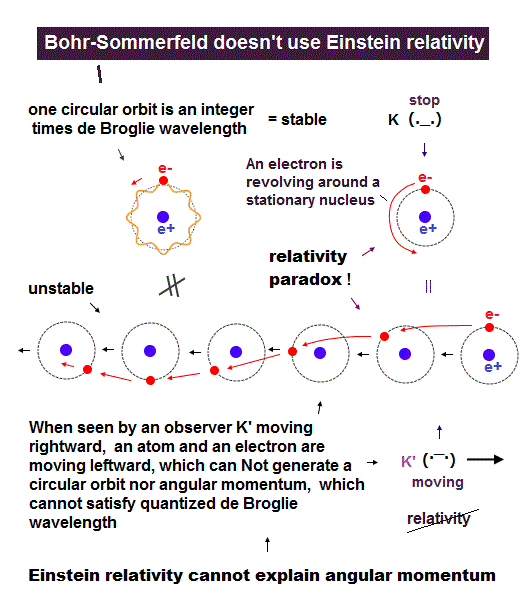The conclusion is that Einstein relativity is wrong, because it's accompanied by various true paradoxes.

The result of believing Einstein wrong theory is unrealistic string theory with fictional 10 spacetime dimensions.

Then why did physicists need to believe Einstein false relativity, even by hiding its true paradoxes ?

The root of all evils is the useless quantum mechanics.  Unless they created artificial relativistic field theory, there would have been nothing to do.

So physicists needed to fabricate "imaginary" targets based on Einstein relativity to attract people's attention and collect money.

That is the truth.2016/2/26 updated. Feel free to link to this site.